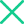# 元素地牢武士技能怎么选 武士技能搭配指南

武士技能

电+水=水遁术

电+土=带电地雷

电+冰=又麻又冻

电+火=火雷爆

电+暗=诅咒斩

冰+土=冻住

冰+火=霜火刀气

冰+暗=真空斩

冰+水=冰刺

土+暗=定身刺

土+水=水泥柱

土+火=烈岩斩

水+火=蒸汽连斩

水+暗=气刃斩

火+暗=暗火斩